# Test: Tangents And Normals

## 10 Questions MCQ Test Mathematics (Maths) Class 12 | Test: Tangents And Normals

Description
This mock test of Test: Tangents And Normals for JEE helps you for every JEE entrance exam. This contains 10 Multiple Choice Questions for JEE Test: Tangents And Normals (mcq) to study with solutions a complete question bank. The solved questions answers in this Test: Tangents And Normals quiz give you a good mix of easy questions and tough questions. JEE students definitely take this Test: Tangents And Normals exercise for a better result in the exam. You can find other Test: Tangents And Normals extra questions, long questions & short questions for JEE on EduRev as well by searching above.
QUESTION: 1

### Find slope of normal to the curve y=5x2-10x + 7 at x=1​

Solution:

y = 5x2 - 10x + 7
dy/dx = 10x - 10
(At x = 1) 10(1) - 10
m1 = 0
As we know that slope, m1m2 = -1
=> 0(m2) = -1
m2 = -1/0 (which is not defined)

QUESTION: 2

### The equation of the tangent line to the curve y =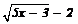which is parallel to the line 4x -2y + 3 = 0 is​

Solution: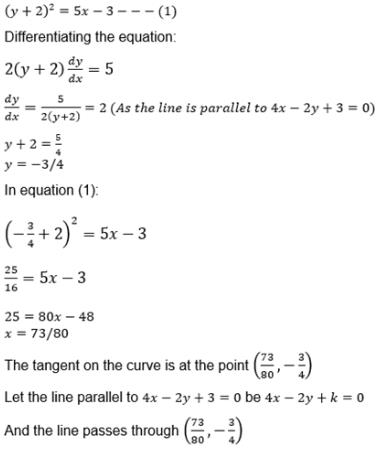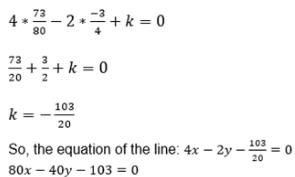QUESTION: 3

### Find the equation of tangent to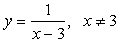which has slope 2.

Solution:

y = 1/(x-3)
dy/dx = d/dx(x-3)-1
dy/dx = (-1) (x-3)(-1-1) . d(x-3)/dx
dy/dx = -(x-3)(-2)
dy/dx = - 1/(x-3)2
Given, slope = 2, dy/dx = 2
- 1/(x-3)2 = 2
⇒ -1 = 2(x-3)2
2(x-3)2 = -1
(x-3)2 = -½
We know that square of any number is always positive So, (x-3)2 > 0
(x-3)2 = -½ is not possible
No tangent to the curve has slope 2.

QUESTION: 4

If y = – sin2x. Find the values of x at which the tangents drawn to the graph of this function is parallel to the x- axis.

Solution:
QUESTION: 5

The normal at any point q to the curve x = a (cos q + q sin q), y = a (sin q – q cos q) is at distance from the origin that is equal to… .​

Solution:

Clearly,  dx/dy =tan q = slope of normal = −cotq
Equation of normal at θ' is y−a(sinq − qcosq) = −cotq(x−a(cosq + qsinq)
= ysinq − asin2q + aqcosqsinq
= −xcosq + acos2q + aqsinqcosq
= xcosq + ysinq = a

QUESTION: 6

The equation of the normal to the curve x2 = 4y which passes through the point (1, 2) is.​

Solution:

h= 4k
slope of normal=−1/(dy/dx) = −2h
equation of normal(y − k)= −2h(x−h)
k = 2 + 2/h(1 − h)
(h2) / 4 = 2 + 2/h (1 − h)
h = 2, k = 1
equation of line (y - 1)= -1(x - 2)
x + y = 3

QUESTION: 7

The curve y = ax3 + bx2 + cx + 5 touches the x-axis at P(-2, 0) and cuts the y-axis at the point Q where its gradient is 3. the equation of the curve is… .

Solution:

y=ax3+bx2+cx+5
(−z,0) lies on curve y=ax3+ bx2 + cx + 5d
⇒ 0= −8a + 4b − 2c + 5.....(1)
Also at (−2,0) curve touches x-axis ie dy/dx at (−2,0) is 0
∴ dy/dx=3ax2+2bx+c
dy/dx (−2,0)=0=12a = −4b + c....(2)
Since curve crosses y-axis at Q, x-coordinate of point Q must be zero
⇒ y = 0 + 0 + 0 + 5
⇒y = 5
∴ coordinate of Q = (0,5)
According to Question  dy/dx =3 at Q(0,5)
⇒ 3= 0 + 0 + c
⇒ c = 3
putting the value of c in eqn(1) & eq n(2)and gurthare solving we get
a=−1/2 & b=-3/4
​∴Eqn of cover is y = −(x3) / 2−3/4x+ 3x + 5

QUESTION: 8

If a, b are real numbers such that x3-ax2 + bx – 6 = 0 has its roots real and positive then minimum value of b is

Solution:

If  p,q  and  r are the roots,
pq + qr + pr = b  and  pqr = 6
Since  A.M.≥G.M . applying it to  3  numbers  pq,qr  and  pr
(pq + qr + pr)/3 ≥ (p2q2r2)1/3  i.e.
(pq + qr + pr)/3 ≥ (36)1/3
pq + qr + pr ≥ 3 (36)1/3
So minimum value of  b  is  (36)1/3

QUESTION: 9

The equation of tangent to the curve y = x3 + 2x + 6 which is perpendicular to the line x + 14y + 4 = 0 is :​

Solution:

y = x3 + 2x + 6
Slope of tangent = m1 = dy/dx = 3x3 + 2
Slope of perpendicular line = m2 = −1/14
m1 . m2  = -1
m1 = 14
3x2 + 2 = 14
x = ±2
Therefore the curve has tangents at x = 2 and x = -2
and these points also lie on the given curve
Equation of tangent - y = 14x + c
Coordinates of points of tangency
At x = 2 , y = 23 + 2(2) + 6 = 18
At x = -2 , y = (-2)3 + 2(-2) + 6 = -6
18 = 14(2) + c
c = -10
-6 = 14(-2) + c
c = 22
Equation of tangent - y = 14x - 10 and y = 14x + 22
Hence, 14x - y +22 = 0

QUESTION: 10

The equations of the tangents drawn to the curve y2 – 2x3 – 4y + 8 = 0 from the point (1, 2) is… .

Solution:

Let P(α, β) be any point on the curve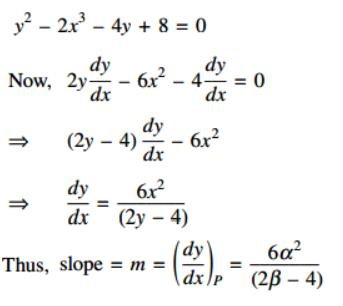Now, the equation of the tangent at P is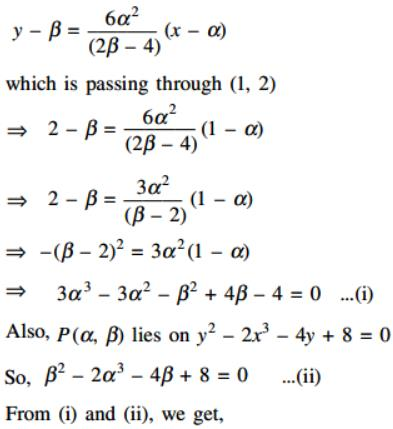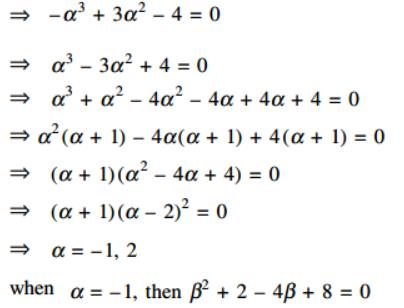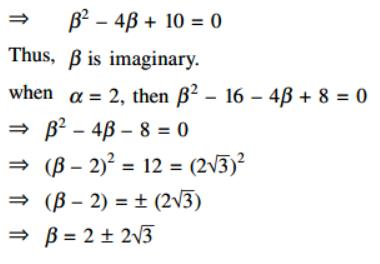Hence, the point of contacts are
(2, 2 + 2√3) and (2, 2 – 2√3)
Slope of the tangents are 2√3 , –2√3
Hence, the equations of tangents are
y – (2 + 2√3) = 2√3 (x – 2)
and
y –(2 – 2√3) = – 2√3 (x – 2)